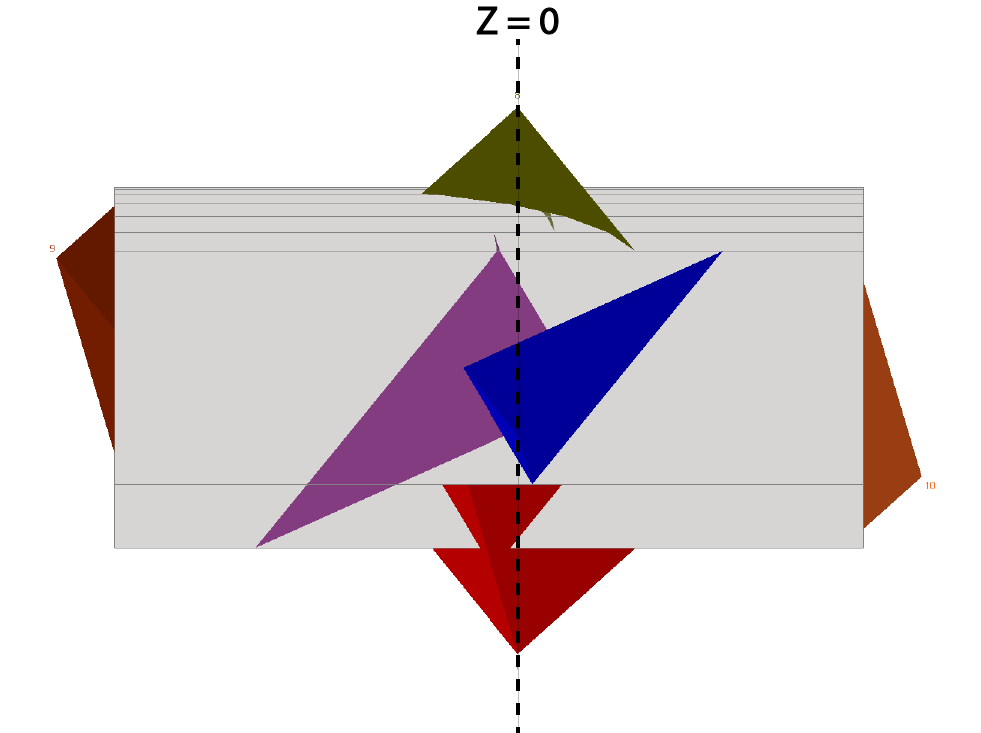The Rocscience International Conference 2021 Proceedings are now available. Read Now

# 3D Coordinates

When you create an UnWedge model, a three-dimensional coordinate system is in effect, where the X, Y coordinates are in the plane of the Opening Section, and the Z-axis coordinates are perpendicular to the Opening Section (i.e., parallel to the tunnel axis).

## Display of Coordinates

In the 3D Wedge View, if the cursor is placed in any of the three orthogonal views (Top / Front / Side), the cursor coordinates are displayed in the status bar at the lower right corner of the application window:

• In the Top view the X , Z coordinates are displayed.
• In the Front view the X, Y coordinates are displayed.
• In the Side view the Z, Y coordinates are displayed.

NOTE: If you use the Length and Angles measurement option, X, Y, Z coordinates are displayed in all views including the Perspective View.

## X , Y Coordinates

The x and y coordinates are based on the coordinates of the Opening Section boundary which you have defined.

## Z Coordinates

The Z-axis coordinates are defined as follows:

• The origin (z = 0) of the z-axis coordinates, corresponds to the apex location of the Perimeter Wedges. In UnWedge, the Perimeter Wedges are displayed along the tunnel length, such that the apex of each Perimeter Wedge, lies in the same plane, parallel to the Opening Section. By definition, this plane is the origin (z = 0) of the z-axis. See the figure below.Side view of UnWedge model showing z-axis origin aligned with apex of Perimeter Wedges.

If you are facing the Opening Section:

• The positive z-axis points toward the viewer (out of the screen)
• The negative z-axis points away from the viewer (into the screen)

NOTE: The z-axis origin (z = 0) is not necessarily at the center of the tunnel length, as it is displayed in UnWedge. For a typical model, z = 0 will probably appear to be at or near the midpoint of the tunnel length. However, if the Perimeter Wedges are asymmetrically distributed around z = 0, then z = 0 will not coincide with the midpoint of the tunnel length. This is simply how the wedges and tunnel are displayed in UnWedge, and has nothing to do with the wedge analysis.